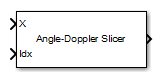# Data Cube Slicer

Slice data cube along specified dimensions

•Libraries:
Phased Array System Toolbox / Space-Time Adaptive Processing

## Description

The Data Cube Slicer block slices a data cube through a specified plane. The input is a data cube of dimensions M-by-Q-by-N. The first dimension is range, or fast time. The second dimension is angle, or channels. The third dimension is Doppler, or slow time.

## Ports

### Input

expand all

Input data cube, specified as an M-by-Q-by-N complex-valued matrix.

Data Types: `double`
Complex Number Support: Yes

Slice-plane index, specified as a positive integer. This index selects which slice to use to create the two-dimensional output.

Data Types: `double`

### Output

expand all

Collapsed data cube, returned as a complex-valued matrix.

• If you set the Output Slice parameter to `Angle-Doppler`, the slice is the constant-range or fast-time plane. The output has dimensions Q-by-N.

• If you set the Output Slice parameter to `Range-Doppler`, the slice is the constant-angle plane. The output has dimension M-by-N.

• If you set the Output Slice parameter to `Range-angle`, the slice is the constant-Doppler plane. The output has dimension M-by-Q.

Data Types: `double`
Complex Number Support: Yes

## Parameters

expand all

Output slice, specified as `Angle-Doppler`, `Range-Doppler`, or `Range-angle`. The output slices are:

Slice planeSlice dimensions
`Angle-Doppler`Q-by-N
`Range-Doppler`M-by-N
`Range-angle`M-by-Q

## Version History

Introduced in R2014b